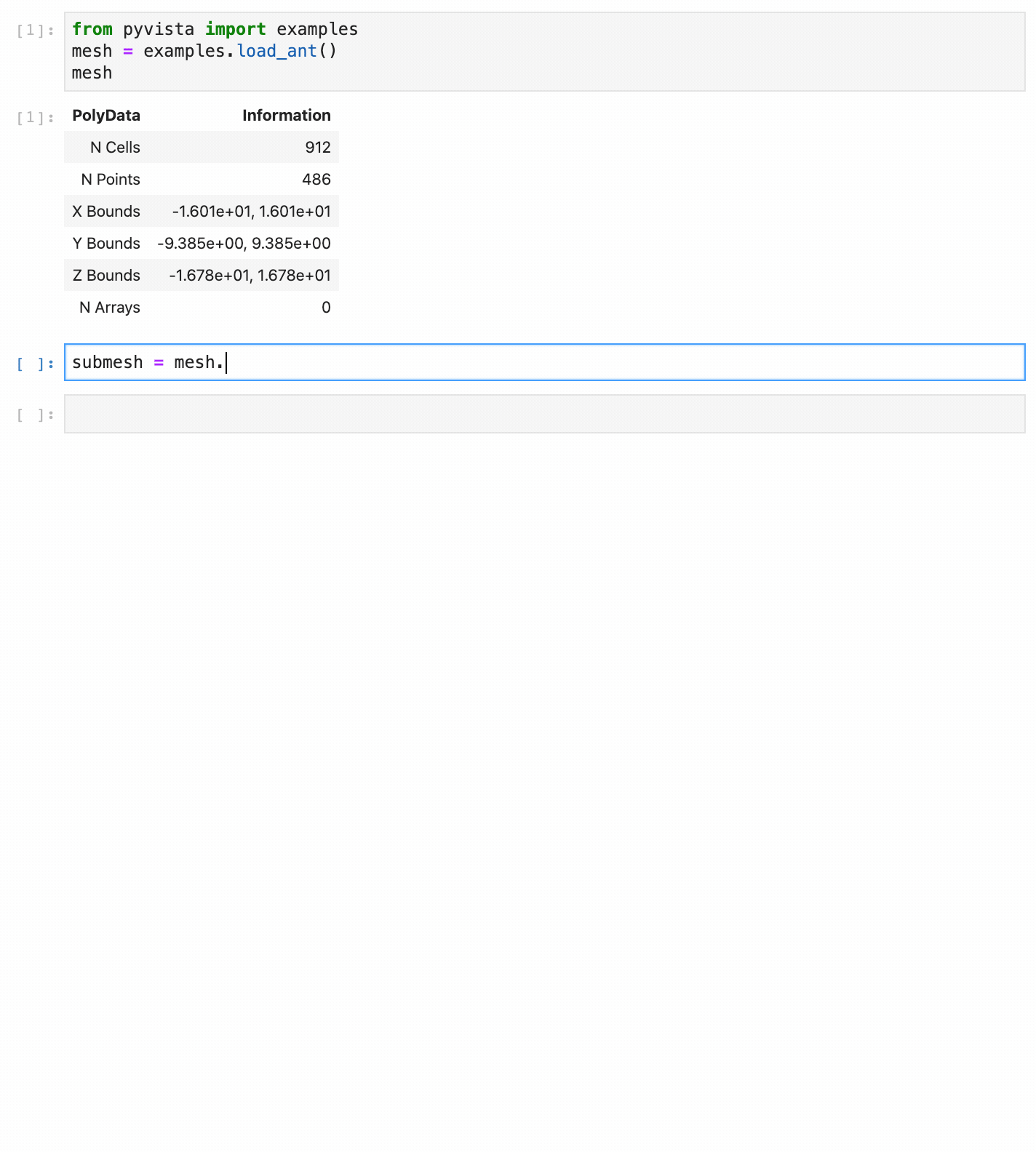# Why PyVista?¶

VTK is an excellent visualization toolkit, and with Python bindings it should be able to combine the speed of C++ with the rapid prototyping of Python. However, despite this VTK code programmed in Python generally looks the same as its C++ counterpart. This module seeks to simplify mesh creation and plotting without losing functionality.

Compare two approaches for loading and plotting a surface mesh from a file:

## Plotting a Mesh using Python’s VTK¶

Using this example, loading and plotting an STL file requires a lot of code when using only the `vtk` module.

```import vtk

mapper = vtk.vtkPolyDataMapper()
if vtk.VTK_MAJOR_VERSION <= 5:
else:

# create actor
actor = vtk.vtkActor()
actor.SetMapper(mapper)

# Create a rendering window and renderer
ren = vtk.vtkRenderer()
renWin = vtk.vtkRenderWindow()

# Create a renderwindowinteractor
iren = vtk.vtkRenderWindowInteractor()
iren.SetRenderWindow(renWin)

# Assign actor to the renderer

# Enable user interface interactor
iren.Initialize()
renWin.Render()
iren.Start()

# clean up objects
del iren
del renWin
```

## Plot a Mesh using PyVista¶

The same stl can be loaded and plotted using pyvista with:

```import pyvista

mesh = pyvista.PolyData('myfile.stl')
mesh.plot()
```

The mesh object is more pythonic and the code is much more straightforward. Garbage collection is taken care of automatically and the renderer is cleaned up after the user closes the VTK plotting window.

When combined with numpy, you can make some truly spectacular plots:

```import pyvista
import numpy as np

# Make a grid
x, y, z = np.meshgrid(np.linspace(-5, 5, 20),
np.linspace(-5, 5, 20),
np.linspace(-5, 5, 5))

points = np.empty((x.size, 3))
points[:, 0] = x.ravel('F')
points[:, 1] = y.ravel('F')
points[:, 2] = z.ravel('F')

# Compute a direction for the vector field
direction = np.sin(points)**3

# plot using the plotting class
plobj = pyvista.Plotter()
plobj.show(screenshot='vectorfield.png')
```While not everything can be simplified without losing functionality, many of the objects can. For example, triangular surface meshes in VTK can be subdivided but every other object in VTK cannot. It then makes sense that a subdivided method be added to the existing triangular surface mesh. That way, subdivision can be performed with:

```from pyvista import examples